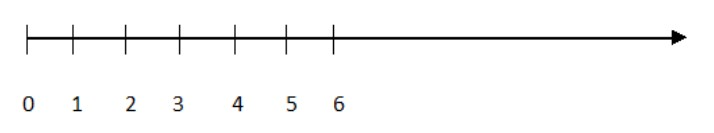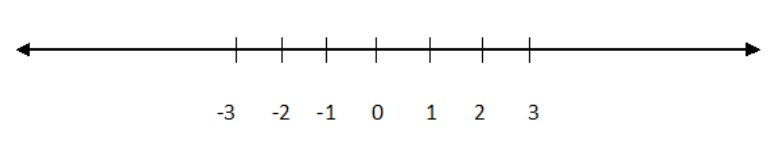Write all the integers between $- 9$ and $3$ which are odd.Verified
146.7k+ views
Hint:We know that, Integers can be negative $\left\{ { - 1,\, - 2,\, - 3,\; \ldots \ldots } \right\}$, positive $\left\{ {1,{\text{ }}2,\,{\text{ }}3,{\text{ }}4,{\text{ }} \ldots \ldots } \right\}$ or zero$\left\{ 0 \right\}$.Also we know that Odd numbers, when divided by $2$, leave a remainder of $1$. So the odd integers are the positive or negative numbers which leave a remainder $1$ when it is divided by $2$.

We need to find out all the integers between $- 9$ and $3$ which are odd.
We know that, Integers can be negative $\left\{ { - 1,\, - 2,{\text{ }} - 3,\,{\text{ }} \ldots \ldots } \right\}$, positive $\left\{ {1,{\text{ }}2,\,{\text{ }}3,{\text{ }}4,{\text{ }} \ldots \ldots } \right\}$ or zero$\left\{ 0 \right\}$.
So the integers between $- 9$ and $3$ are $\left\{ { - 8,{\text{ }} - 7,\, - 6,{\text{ }} - 5,\; - 4,\; - 3,{\text{ }} - 2,\; - 1,\,0,{\text{ }}1,\,2} \right\}$
Now we need to find out the odd numbers from the set $\left\{ { - 8,{\text{ }} - 7,\, - 6,{\text{ }} - 5,\; - 4,\; - 3,{\text{ }} - 2,\; - 1,\,0,{\text{ }}1,\,2} \right\}$.
That is which leaves a remainder $1$ when divided by $2$.
That is the odd numbers from the set $\left\{ { - 8,{\text{ }} - 7,\, - 6,{\text{ }} - 5,\; - 4,\; - 3,{\text{ }} - 2,\; - 1,\,0,{\text{ }}1,\,2} \right\}$ can be derived by finding the numbers which leave a remainder $1$ when it is divided by $2$.
They are $- 7,{\text{ }} - 5,\; - 3,\, - 1,\;1$.
Therefore all the integers between -9 and 3 which are odd are $- 7,{\text{ }} - 5,\; - 3,\, - 1,\;1$.

Note:
Whole numbers:
Whole numbers are simply the numbers in which zero is included $0,{\text{ }}1,{\text{ }}2,{\text{ }}3,\;{\text{ }}4,\,{\text{ }}5,{\text{ }}6,{\text{ }} \ldots ..$Integers:
Integers are like whole numbers, but they also include negative numbers but still no fractions allowed.So integers can be negative $\left\{ { - 1,{\text{ }} - 2,{\text{ }} - 3,\; \ldots \ldots } \right\}$, positive $\left\{ {1,\,{\text{ }}2,\,{\text{ }}3,{\text{ }}4,{\text{ }} \ldots \ldots } \right\}$ or zero $\left\{ 0 \right\}$.